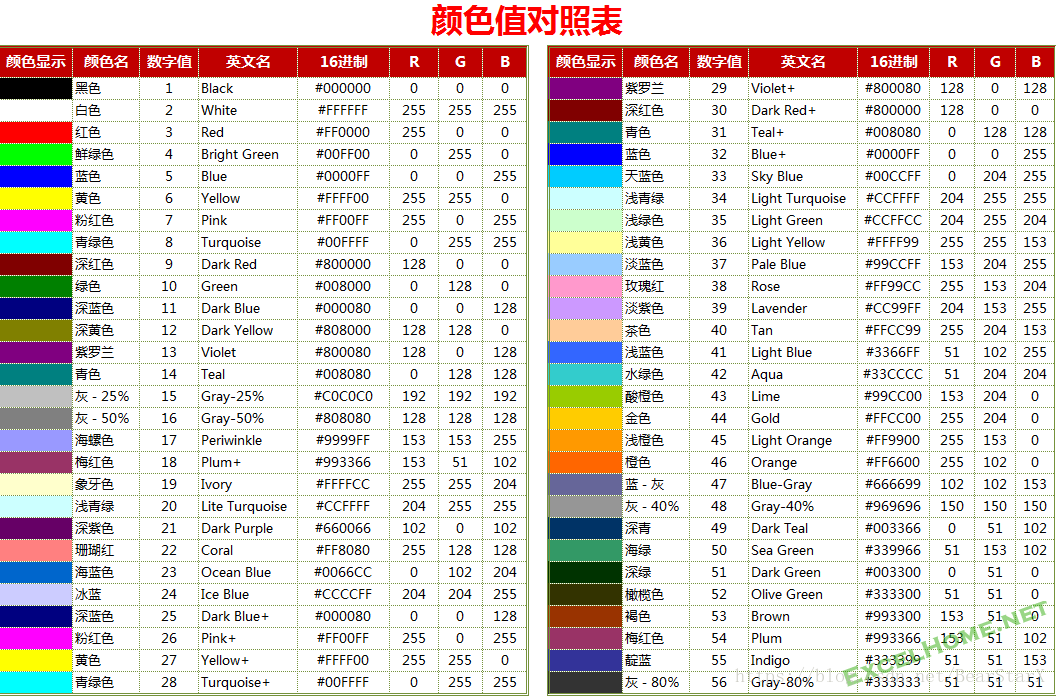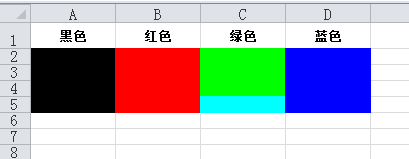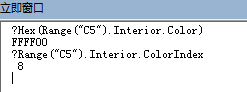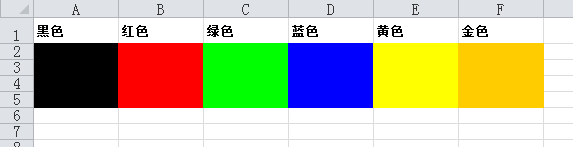# 【VBA研究】关于单元格颜色值的十六进制赋值

113 篇文章 12 订阅

1、单元格颜色的设置RGB(R,G,B)=B*256*256+G*256+R

&HBBGGRR，其中RR、GG、BB是红绿蓝三种颜色十六进制值。

2、颜色设置方法

3、颜色设置代码和效果

'设置单元格背景颜色
Sub setColor()
'黑色
Range("A1") = "黑色"
Range("A2").Interior.ColorIndex = 1
Range("A3").Interior.Color = RGB(0, 0, 0)
Range("A4").Interior.Color = 0
Range("A5").Interior.Color = &H0
'红色
Range("B1") = "红色"
Range("B2").Interior.ColorIndex = 3
Range("B3").Interior.Color = RGB(255, 0, 0)
Range("B4").Interior.Color = 255
Range("B5").Interior.Color = &HFF
'绿色
Range("C1") = "绿色"
Range("C2").Interior.ColorIndex = 4
Range("C3").Interior.Color = RGB(0, 255, 0)
Range("C4").Interior.Color = 65280      '255*256=65280
Range("C5").Interior.Color = &HFF00
'蓝色
Range("D1") = "蓝色"
Range("D2").Interior.ColorIndex = 5
Range("D3").Interior.Color = RGB(0, 0, 255)
Range("D4").Interior.Color = 16711680   '255*256*256=16711680
Range("D5").Interior.Color = &HFF0000
End SubRange("C5").Interior.Color = &HFFFF00

效果完全相同。

Range("C5").Interior.Color = &H100FF00 - &H1000000

VBA没有无符号整数，所以用VBA处理无符号整数（比如移位），会碰到与上面类似的问题，这一点要注意。真要处理，就用Byte类型吧。

Sub setColor()
'黑色
Range("A1") = "黑色"
Range("A2").Interior.ColorIndex = 1
Range("A3").Interior.Color = RGB(0, 0, 0)
Range("A4").Interior.Color = 0
Range("A5").Interior.Color = &HCC000000
'红色
Range("B1") = "红色"
Range("B2").Interior.ColorIndex = 3
Range("B3").Interior.Color = RGB(255, 0, 0)
Range("B4").Interior.Color = 255
Range("B5").Interior.Color = &HCC0000FF
'绿色
Range("C1") = "绿色"
Range("C2").Interior.ColorIndex = 4
Range("C3").Interior.Color = RGB(0, 255, 0)
Range("C4").Interior.Color = 65280     '255*256
Range("C5").Interior.Color = &HCC00FF00
'蓝色
Range("D1") = "蓝色"
Range("D2").Interior.ColorIndex = 5
Range("D3").Interior.Color = RGB(0, 0, 255)
Range("D4").Interior.Color = 16711680     '255*256*256
Range("D5").Interior.Color = &HCCFF0000
'黄色
Range("E1") = "黄色"
Range("E2").Interior.ColorIndex = 6
Range("E3").Interior.Color = RGB(255, 255, 0)
Range("E4").Interior.Color = 65535     '255*256+255
Range("E5").Interior.Color = &HCC00FFFF
'金色
Range("F1") = "金色"
Range("F2").Interior.ColorIndex = 44
Range("F3").Interior.Color = RGB(255, 204, 0)
Range("F4").Interior.Color = 52479     '204*256+255
Range("F5").Interior.Color = &HCC00CCFF

End Sub11-153928
04-061万+11-224088
12-1477
03-23225
09-078509
11-273649
08-069944
05-102万+
10-04923
01-311011
07-21774
02-07240
03-076万+
09-258427
06-121055
03-24152
06-141447
06-14699点击重新获取扫码支付余额充值